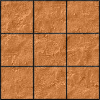#### You may also like### Pyramids

What are the missing numbers in the pyramids?### Paving the Way

A man paved a square courtyard and then decided that it was too small. He took up the tiles, bought 100 more and used them to pave another square courtyard. How many tiles did he use altogether?### Chess

What would be the smallest number of moves needed to move a Knight from a chess set from one corner to the opposite corner of a 99 by 99 square board?

# Reversals

##### Age 11 to 14 Challenge Level:

You may wish to try Always a Multiple? and Special Numbers before tackling this problem.

Where should you start, if you want to finish back where you started?

Alison chose a two-digit number, divided it by $2$, multiplied the answer by $9$, and then reversed the digits.
Her answer was the same as her original number!
Can you find the number she chose?

Then she chose another two-digit number, added $1$, divided the answer by $2$, and then reversed the digits.
Again, her answer was the same as her original number!
Can you find the number she chose this time?

Charlie chose a two-digit number, subtracted $2$, divided the answer by $2$, and then reversed the digits.
His answer was the same as his original number!
What was Charlie's number?

Choose a number, subtract $10$, divide by $2$ and reverse the digits.

Extension

Choose a 3-digit number where the last two digits sum to the first (e.g. $615$).

Rotate the digits one place, so the first digit becomes the last (so for the example, we get $156$).

Subtract the smallest number from the largest and divide by $9$ (which is always possible).

What do you notice about the result? Can you explain why?

With thanks to Don Steward, whose ideas formed the basis of this problem.